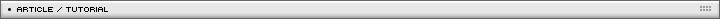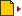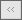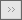Case study: building a puzzle game using AS3 and OOP Page 1/1[ November 10, 2007 ] Eitan Avgil Learn how to create a simple "match the colors" puzzle game using OOP techniques in Actionscript 3

The game is a simple color match game. The goal is to order all colors by the color of the left column. Right now there are up to 6 colors but it can be easily extend to n colors.

The idea behind this simple game's logic is simple. Clicking one button – will check if it's near the empty place, and if it is – it will move to the empty place coordinates. This looks like there is an empty place there but actually there is a regular square – same class as all squares with a special color and no interactivity. The switching has no animation but because the squares are so close it looks like they are moving. Once all squares are in position the timer is stopped.

We have 4 objects (4 classes) in this game:

• Main class
• Matrix
• Logic
• Box

Main class

The main class is responsible of the following tasks:

• create a Matrix instance, passing its parameters;
• create a Logic instance, passing it the array from the Matrix and the WhiteBox;
• make the AddChild of the Matrix;
• create and manage the textField and the timer.
```package {
import flash.display.Sprite;
import flash.text.TextField;
import flash.events.MouseEvent;
import flash.events.Event;
import flash.utils.Timer;
import flash.events.TimerEvent;
/**
* main application. calls the matrix and send rows & columns
* creates logic. set 'start' button and set timer & textfield;
* @author Eitan Avgil
*
*/
public class Main extends Sprite
{
private var _matrix:Matrix;
private var _tf:TextField;
private var _logic:Logic;
private var _timer:Timer;

public function Main()	{
_matrix = new Matrix(3,5);
_matrix.x = 60;
_matrix.y = 80;
_tf = new TextField();
_tf.border = true;
_tf.height = 20;
_tf.x = 10;
_tf.y = 10;
_tf.width = 150;
_tf.selectable = false;

var startButton:Sprite = new Sprite();
startButton.graphics.beginFill(0xCCCCCC)
startButton.graphics.drawRect(0,0,50,20)
var tf:TextField = new TextField()
tf.text = "start";
tf.selectable = false;
startButton.x = 50;
startButton.y = 50;

}
/**
* start the game by clicking the start text
* @param evt
*
*/
private function onClick(evt:MouseEvent):void{
removeChild(evt.currentTarget as Sprite);
_logic = new Logic(_matrix.boxesArray,_matrix.whiteBox);

_timer = new Timer(10);
_timer.start()
}
/**
* end game text
* @param evt
*
*/
private function onGameEnd(evt:Event):void{
_timer.stop();
_tf.text = "DONE >> "+numberToTime(_timer.currentCount)
}

/**
* timer counter
* @param evt
*
*/
private function onTimerTic(evt:TimerEvent):void{
_tf.text = numberToTime(Number(Timer(evt.target).currentCount));
}

/**
* rounds the timer to 2 decimal after point
* @param n
* @return
*
*/
private function numberToTime(n:uint):String{
return String(n/100);
}
}
}
```

Box class

This class represent the single square. The class gets a color code parameter in its constructor and has to draw a square with this color. Once this square is clicked, the instance dispatches an event. The class that manages all boxes will catch this event and will know what to do with it.
Some boxes are not interactive (the whitebox and the left column boxes) so they will get a special treat by removing their click dispatch. If we would make this a perfect OOP model, these instances would be an extension of the Box class, but I decided that this project would show how to break a game to classes and object and did not want to add too much OOP.

Each Box gets (through a setter function) its initial ycoordinate, so it will be "able", when asked, to tell us if it is sitting in the correct row or in a wrong row.

These are the public methods of the square:

• Constructor – gets a color code, transparency and border thickness
• set heightInit – to set the init Y coordinate
• setEmptyBox – to set the whitebox
• disableInteractive – remove the mouseEvent listening if this box is not interactive
• isInOriginalHeight – box self check if it is in the correct row (returns true/false)

The class also holds a static const of the square width, and a static event name.

```package
{
import flash.display.Sprite;
import flash.events.MouseEvent;
import flash.events.Event;
/**
* a single square. has no logic. dispatches click event when clicked - thats it.
* @author Eitan Avgil
*
*/
public class Box extends Sprite {

// this height value is here so the box will "know" if it is in the right row
private var _originalHeight:Number;
private var _colorCode:uint;
private var _borderAlpha:Number;
private var _borderWidth:Number;
//color array - matches code to a constant color code
private const COLOR_ARRAY:Array = [0xFF0000,
0x00FF00,
0x0000FF,
0xDD00DD,
0X00FFFF,
0xFFFF00];
// defenition of box size
public static const BOX_SIZE:uint = 20;
//clicked string event name
public static const BOX_CLICKED:String = "clicked";

//C'tor
public function Box(colorCode:uint,borderAlpha:Number=0,borderWidth:Number=0){
_colorCode = colorCode;
_borderAlpha = borderAlpha;
_borderWidth = borderWidth;
drawRect(COLOR_ARRAY[_colorCode]);
}
// just draw graphic rectangle
private function drawRect(color:uint):void{
graphics.beginFill(color);
graphics.lineStyle(_borderWidth,0xFFFFFF,_borderAlpha)
graphics.drawRect(0,0,BOX_SIZE,BOX_SIZE)
graphics.endFill();
}
//inner click listening
private function onMouseClick(evt:MouseEvent):void{
// dispatches event to whome it may listen
//trace(Box(evt.target).colorCode)
dispatchEvent(new Event(BOX_CLICKED));
}

// remember the initialized Y coordinate for checking later on.
public function set heightInit(value:Number):void{
_originalHeight = value;
}

// the whiteBox
public function setEmptyBox():void{
graphics.clear();
_colorCode = undefined;
drawRect(0xFFFFFF);
alpha=0.2;
}
//remove interactive for the non-interactive boxes
public function disableInteractive():void{
removeEventListener(MouseEvent.CLICK,onMouseClick);
}
// check if current instance is in it's initialize (correct) Y coordinate.
public function isInOriginalHeight():Boolean{
if(this.y==_originalHeight) return true;
return false
}
}
}```

Matrix class

This class has a single instance in the game, and it creates and arrange all boxes. Its responsibility is to mix all squares (only interactive squares) and let other classes get the array of all boxes: create, arrange mix and gather all boxes in an array. That's it.
We could also write the logic part of the game in it, but we wanted to show how to divide the creation and the logic, so we decided to make 2 separated classes.

Matrix class has the following public methods:

• Constructor – gets rows and columns numbers
• get boxesArray – return an array of all interactive boxes
• get whiteBox – returns the instance of the whitebox

Although the shuffling function is a private method, we would like to say a thing or two about it. The main idea of the shuffling here is swapping objects coordinates. No array commands and no 2 dimensions array are involve here. So, how is it done?
When we create the boxes, we push them into an array (simple one dimention array) in the order we create them. The mixing function runs on the array, takes the instance of the current index and just replace its coordinates with those of another random box from the array. In this way, every box is being replaced at least once. All boxes are in the array, only in mixed coordinates.

```package
{
import flash.display.Sprite;
import flash.geom.Point;
/**
* a visual class - places boxes by rows & columns
* places the empty box
* scramble all boxes
* @author Eitan Avgil
*/
public class Matrix extends Sprite{

private var _whiteBox:Box
private var _rows:uint;
private var _cols:uint;
private var _gap:uint; // space between the boxes
private var _boxes:Array; // 2 dimentions array of organized colored boxes
public static const GAP:uint = 5;

public function Matrix(rows:uint,cols:uint){
_rows = rows;
_cols = cols;
_boxes = new Array();
buildMatrix();
buildGuideBoxes();
scramble();
}
// build the matrix. and pushes all boxes to an array
private function buildMatrix():void{
var i:uint;
var j:uint;
var box:Box;
for(i=0;i< _rows;i++){
for(j=0;j< _cols;j++){
// create a new box and push it to the row
box = new Box(i);
box.x = j*(Box.BOX_SIZE+GAP);
box.y = i*(Box.BOX_SIZE+GAP);
box.heightInit = box.y;
_boxes.push(box);
}
}
}
// build the left collumn boxes
private function buildGuideBoxes():void{
var j:uint;
var box:Box;
for(j=0;j< _rows;j++){
box = new Box(j,1,2);
box.disableInteractive();
box.x = -Box.BOX_SIZE-(GAP*3);
box.y = j*(Box.BOX_SIZE+GAP);
}
}
// creates the non-colored box
_whiteBox = new Box(0);
_whiteBox.setEmptyBox();
_whiteBox.disableInteractive();
_whiteBox.y = -Box.BOX_SIZE-GAP;
_whiteBox.heightInit = _whiteBox.y;
}

// scramble all boxes. make sure whitebox and first box is nor scrambled
private function scramble():void{
var point:Point
var box:Box;
var tmpBox:Box
var rnd:uint;
var max:uint = _boxes.length-1;
for (var i:uint=1;i< max;i++){
box = _boxes[i];
point = new Point(box.x,box.y);
rnd=(Math.random()*max)+1;
tmpBox = _boxes[rnd];
switchPlaces(box,tmpBox);
}
}

// switches places between 2 boxes
private function switchPlaces(box:Box,box2:Box):void{
var point:Point = new Point(box.x,box.y);
box.x = box2.x;
box.y = box2.y;
box2.x = point.x;
box2.y = point.y;
}

public function get boxesArray():Array{
return _boxes;
}

public function get whiteBox():Box{
return _whiteBox;
}
}
}```

Logic class

This class is the brain of the game. It gets an array (the array created by the matrix class) and just listen to events from its elements. After adding the eventListeners it sits and waits that one of the boxes dispatches the "I was clicked" event.
When the Logic class gets this event, it checks if the box that dispatched the event is a box next to the whitebox, by checking its coordinates. It checks if they have both (clicked box and whitebox) the same Y coordinate and if so, if the horizontal offset between them is equal to one box width (plus the gap); same thing for the other dimension.
If, and only if, the clicked box is next to the whitebox we switch their coordinates. After switching them, the Logic class checks if the whitebox is in its original place (out of the colored rows) and if yes, it checks all boxes positions. We divided this check in two parts because we don't need to check on each switch if all boxes are in place. The game can be over only when the whitebox is in its place, so that way we can save unnecessary checks.
The check of all boxes is very simple. Because we gave each box the ability to "know" if it is in the correct Y coordinate, all we have to do now is just a simple for loop and just ask each box "are you ok there?". If each box wouldn't know if it is placed correctly, the checking would be a bit more complex (what is your color? what is your Y position? is this position right for this color?).

A better OOP designed game would separate the view from the data, so the check would be simple on the data side, but this is too OOP for this small game.

```package
{
import flash.events.EventDispatcher;
import flash.events.Event;
import flash.geom.Point;

public class Logic extends EventDispatcher{

private var _boxes:Array;
private var _whiteBox:Box;

//C'tor
public function Logic(boxes:Array,whiteBox:Box){
_boxes = boxes;
_whiteBox = whiteBox;
}

// add click listener to the interactive boxes.
var box:Box;
for(var i:uint=0;i< _boxes.length;i++){
box = _boxes[i] as Box;
}
}

// the function that is attached to clicks on every interactive box
private function onBoxClicked(evt:Event):void{
var box:Box = evt.currentTarget as Box;
checkBox(box);
}

// checks if the given box is next to the whiteBox
private function checkBox(box:Box):void{
if((box.x==_whiteBox.x && (Math.abs(box.y-_whiteBox.y)==Box.BOX_SIZE+Matrix.GAP))
|| (box.y==_whiteBox.y && (Math.abs(box.x-_whiteBox.x)==Box.BOX_SIZE+Matrix.GAP))){
switchPlaces(box,_whiteBox);
}
}

//switches places between the white box and a given box
private function switchPlaces(box:Box,box2:Box):void{
var point:Point = new Point(box.x,box.y);
box.x = box2.x;
box.y = box2.y;
box2.x = point.x;
box2.y = point.y;
checkWhiteBox();
}

// check if the whitebox is in it's place. only if it is -
// continue checking all other boxes.
private function checkWhiteBox():void{
if(_whiteBox.isInOriginalHeight()){
//trace("whitebox in place - let's check all other boxes")
checkAllBoxes();
}
}

// ask each box if it is in it's original height
private function checkAllBoxes():void{
for (var i:uint=0;i< _boxes.length;i++){
if(!Box(_boxes[i]).isInOriginalHeight()){
//trace("sorry - not all boxes are in their places");
return;
}
}
//trace("all in place")
dispatchEvent(new Event(Event.COMPLETE));
}

}
}```

Summary

This application was built to show some OO principles:

• how to break the application into different objects, and the responsibility of each object;
• how to build a visual matrix and mix the boxes;
• how to pass informations (array of boxes) and separate the logic layer and the visual layer;
• how to establish communication between classes: lower class shoots events to higher class, higher class use direct access through instance pointer;
• how static elements are used between classes (events names, constant numbers).

Here's the Adobe Flex project files packed in one sigle rar: Boxes.rarName: Eitan Avgil Location: Israel Age: 32 Flash experience: About 5 years experience in flash programming, 2 of them in OOP environment; about one year in AS3. Runs a flash community (in hebrew) called Flashoo and an AS3 blog. Job: Team Leader of a small game development team Website: http://www.avgil.com/| Homepage | News | Games | Articles | Multiplayer Central | Reviews | Spotlight | Forums | Info | Links | Contact us | Advertise | Credits || www.smartfoxserver.com | www.gotoandplay.biz | www.openspace-engine.com | gotoAndPlay() v 3.0.0 -- (c)2003-2008 gotoAndPlay() Team -- P.IVA 03121770048